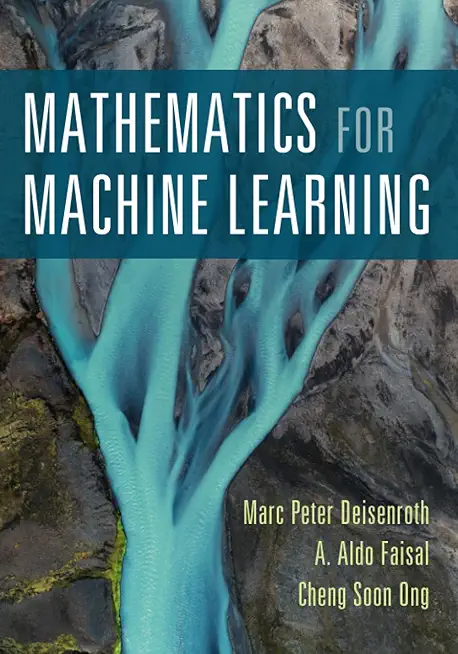### Mathematics for Machine Learning### Huge savings for students

Each student receives a 50% discount off of most books in the HSG Book Store. During class, please ask the instructor about purchase details.
 List Price: \$46.99 Price: \$23.50 You Save: \$23.50
4The fundamental mathematical tools needed to understand machine learning include linear algebra, analytic geometry, matrix decompositions, vector calculus, optimization, probability and statistics. These topics are traditionally taught in disparate courses, making it hard for data science or computer science students, or professionals, to efficiently learn the mathematics. This self-contained textbook bridges the gap between mathematical and machine learning texts, introducing the mathematical concepts with a minimum of prerequisites. It uses these concepts to derive four central machine learning methods: linear regression, principal component analysis, Gaussian mixture models and support vector machines. For students and others with a mathematical background, these derivations provide a starting point to machine learning texts. For those learning the mathematics for the first time, the methods help build intuition and practical experience with applying mathematical concepts. Every chapter includes worked examples and exercises to test understanding. Programming tutorials are offered on the book's web site.
Cambridge University Press